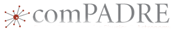# Lab: Horizontally Launched Projectiles (with uncertainty analysis)This material was originally developed through comPADRE
as part of its collaboration with the SERC Pedagogic Service.

## Summary

This is a version of the time-tested lab where students roll a ball off a table top and use kinematics in two dimensions to try to predict where the ball will land. While many versions of this lab have been previously published, in this version students determine the uncertainty of all measurements and uncertainty of their prediction. The techniques and vocabulary are consistent with the Introduction to Measurement packet.

In most versions of this lab, students predict the distance away from the table where they think the ball will land. Then, students launch the projectile to see whether their prediction is right. In this version, students use the concept of uncertainty and predict a range of distances between which they predict the ball will land. Then they launch the projectile to see whether their prediction is correct within their stated range of certainty.

## Learning Goals

The goals for this lab:
• Students will apply kinematics in two dimensions to predict the range of a horizontally launched projectile.
• Student will use effective measurement technique consistent with the Introduction to Measurement (advanced high school/intro college level)
• Students will present the results of their prediction as a range of possible values, based on the uncertainty of their measurements.

## Context for Use

This lab is intended to be used for introductory level high school or college physics students while studying kinematics in two dimensions. It is intended to be used along with other methods of instruction on kinematics. This lab is included here as an example of a commonly used physics lab into which effective measurement technique has been integrated.

## Teaching Notes and Tips

Expect one full hour of work to set up apparatus, and to measure the speed at which the ball will leave the table. Assign the prediction as homework, and allow half an hour for students to test their prediction. Questions can be done as homework after students test their predictions.

## Assessment

Questions in the lab instructions can be used for assessment.